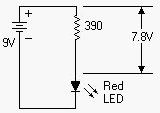Mechanical Television & Illusion Generators by James T. Hawes, AA9DT
Ohm's Law is Easy, Part 1• LED: Use red, orange or yellow.
• Battery: 9-volt transistor battery.
• Resistor: 390-ohm, half-watt.

Resistance ohms (R) are simple. They're the ratio of voltage (E) to current (I). Another way to say the same thing is that volts equal ohms times current.

If E / I = R,
then E = I * R.

You can prove Ohm's Law. See the diagram at right. Attach a 9-volt battery to one end of a 390-ohm, half-watt resistor. Wire the resistor's other end to one lead of a red LED. Touch the LED's loose end to the battery. If the LED doesn't turn on, reverse battery connections.

When the LED turns on, it draws about 0.02 amps from the battery. The resistor drops 7.5 volts to protect the LED. That is, the resistor has some 7.5 volts across it. The LED only needs 1.5 volts. The resistor delivers only what the LED needs. Without the resistor, the LED would melt! You calculate the actual drop across the resistor by multiplying...

390 ohms * 0.02 amps = 7.8 volts
See how easy that is?Web www.hawestv.com

Go to Page:   1   2   3   Next